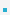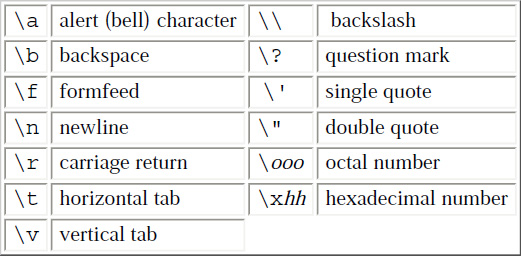4. Constants

Constants are literal/fixed values assigned to variables or used directly in expressions.
 Datatype example meaning integer int x = 3; integer long x = 3L; long integer unsigned long x = 3UL unsigned long Floting-point double x = 3.14; float x = 3.14F; float double x = 3.14L; long double octal or hexadecimal int x = 0x1A hexadecimal int x = 0x37 Octal character ‘\xb’ ASCII vertical tab ‘\x7’ ASCII bell character string “hello, world” string “hello, “ “world” same as “hello, world” because string constants can be concatenated at compile time. enumeration enum BOOL {NO, YES} NO = 0, YES = 1 enum COLOR {R=1, G, B, Y=10} G = 2, B = 3The complete set of escape sequence is이전페이지 / 5 / 다음페이지